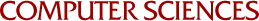The Maximum K-Colorable Subgraph Problem

Giri Narasimhan
1989

For a given graph, the Maximum k-Colorable Subgraph Problem is the problem of determining the largest set of vertices of the graph that can be colored using k distinct colors such that adjacent vertices, if colored, are assigned different colors. This problem is NP-hard for general graphs. We examine several approaches to deal with the hardness of the maximum k-colorable subgraph problem. One of our main results is that algebraic techniques can be used to efficiently compute bounds for the size of the maximum k-colorable subgraph in a graph. As a first approach, we designed polynomial-time algorithms for the maximum 2-colorable subgraph problem on various special classes of graphs like interval graphs, circular-arc graphs, and tolerance graphs. As a second approach, we considered approximation algorithms for the maximum k-colorable subgraph problem. We showed that no good approximation algorithm is possible for general graphs, and we analyzed the naïve greedy approximation algorithm on some special classes of graphs. As a last approach, we used algebraic techniques to design algorithms that find upper bounds for the size of the maximum k-colorable subgraph. This approach was motivated by work due to Laszlo Lovasz. Lovasz proved a sandwich theorem by defining an efficiently computable function of the graph whose value, for any graph, always lies between two interesting, but hard to compute, graph parameters. We proved a generalization of Cloviss sandwich theorem by defining another efficiently computable graph function whose value always lies between two other interesting graph parameters that are hard to compute. One of the bounding parameters is the size of the maximum k-colorable subgraph in a graph, thus providing efficiently computable bounds for this graph parameter.